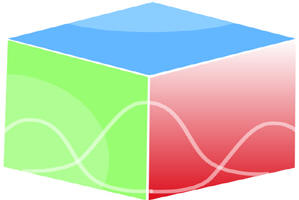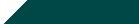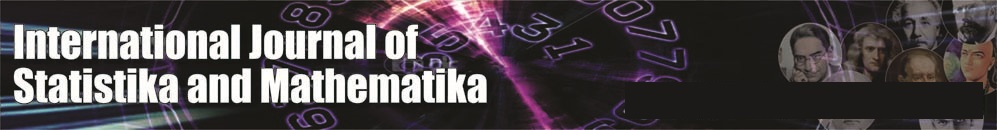``Home| Journals | Statistics Online Expert | About Us | Contact UsUntitled Document

Analysis of Traffic Accident Frequency and Reliability Using Appropriate System Reliability Model - A Simulation Approach

Sujatha Inginshetty

Department of Statistics, Gulbarga University, Gulbarga-585106, Karnataka, INDIA.

[email protected]

Research Article

Abstract: This paper studies the application of system reliability theory with certain modifications to a four lane highway. It can be modeled the four lane highway as a series parallel system. To analyze traffic accident frequency and reliability of each Lane, we generated number of accidents in each section by using simulation technique. Assuming that the time between two accidents will follow an exponential distribution, we determined reliability and accident frequency of each Lane along with its sections. Based on the results obtained, conclusions were drawn.

Key words: Reliability, unreliability, Accident frequency rate, Mean time between two accidents, Poisson process Exponential failure law.

1. Introduction

2. Model

In determining the reliability of a highway, a preliminary analysis is necessary that would determine its structure and level of complexity or defining the system units.

For the analysis, we consider the following assumptions:

1. Assume that a four lane highway-road as a system.

2. Assume that each road (lane) as a sub-system.

3. Assume that each road is divided into n-sub sections with different length based on the distance between two stages and considered these sub sections as units within each subsystem. Thus each road as a complex system consists of n- units that refer sub sections. The section represents road between two stages along with road distance.

4. Assume that a traffic flow interruption in any unit causes the traffic flow interruption    in the      whole road.

5.  Let be the ith unit(section) reliability in jth subsystem(lane), for i=1,2,…..….n  and j=1,2,3,4.

6. Let the Process {N (t), t=1, 2…} be the number of accidents occurs per unit time t and which follows the poison process with its rate parameter λ.

7. Let the time between two accidents follows an exponential distribution with failure rate λ.

8. Let the distribution function of an exponential accident (failure) law is  (2.1)

9.  The diagrammatic representation of the four lane road is as follows:

Fig1: Representation of the four lane road as parallel series system.

In order to obtain an adequate mathematical model with the real system reliability theory, different statistical distributions are approximated with the help of real process. For example the distribution of time between two events is approximated to an exponential failure law. Traffic flow begins at the time t=0 and the time period without traffic accident is interval [0, t1], Where t1 is the moment of occurrence of the first traffic accident. The next period without accident is the interval [t1, t2], where t2 is the moment of occurrence of second traffic accident. In most of the analysis, the Poisson’s distribution of events in stochastic process is used as a starting distribution. The basic form of Poisson distribution is continuous time counting process which possesses the following properties: independent increments, stationary increments, no counted simultaneously occurrences. A homogeneous poisson processes characterized by its rate parameter  which is the expected number of events that occurs per unit time and per Km (Km-Kilometere). If the system of events has poisson distribution, then the time between two occurrences of events (accidents) will follows an exponential distribution with parameter . Therefore it has been adopted that all the empirical distributions of the duration of the time period without accidents can be replaced by the corresponding exponential distribution. The road safety indices like reliability, accident frequency rate and Mean Time Between two Accidents(MTBA) can be determined based on the assumption that the inter arrival time (time between two accident occurrence)  in the ith section is distributed according to an exponential failure(accident) law with the distribution function

(3.1)

Then the probability density function is given by

, where      (3.2)

The distribution function Fi(t) of the random variable T is equal to the probability that an accident will occur before the time t. This function is also called the function of unreliability. The reliability function Ri(t)=1- Fi(t).

The mean time between two consecutive accidents (MTBA) is obtained as

(3.3)

The accident frequency  also represents an important and widely used reliability characteristic and represents the measure of current speed of the accidents occurrence.  can be expressed in various time units and depends on the time units which is used for calculation of MTBA.

For Poisson distribution, the accident frequency as the current speed of the accidents occurrence is constant at the moment in the ith observed time period.

The accident frequency function on the ith section is

(3.4)

The expected number of accidents in a certain period is:

(3.5)

Here we consider the time units as t-168 (hours). The road reliability is determined by the reliability of the sections of that road. The observed road represents the simple series system because all the   sections are in series. Therefore the jth road reliability is

(3.6)

Since all the four lanes (roads) are in parallel, the entire four lanes road reliability is the function of reliability of all the four lane roads. Thus the entire four lane road (system) reliability is

(3.7)

4. Results and conclusions

Obtaining the number of accidents in a particular section or on the road over a longer period of time is practically difficult task as it involves loss of lives, loss of machinery, cost etc. Thus the number of accidents based on the Poisson process can be generated by using simulation technique. In this section, based on the simulated results we determine the reliability of each section -using equation (3.2), each lane (road)-using equation (3.6) and the entire system reliability-using equation (3.7).

Table 1: Representation of observed number of accidents in Lane-I and some appropriate results for analysis

 Lane I Sections (km) Length Observed number of Accidents λi(h-1) T0i(h) Ai(t) Ri(t) 1 40 14 0.002083 480 0.35 0.70468809 2 36 09 0.001488 672 0.25 0.77880078 3 50 15 0.001786 560 0.30 0.74081822 4 20 04 0.001190 840 0.21 0.81873075 5 14 03 0.001276 784 0.22 0.80711774 160 45 0.0078238* 127.83* 1.32* 0.26866615*

*represents the respected characteristics of the Lane-I

Table 2: Representation of observed number of accidents in Lane-II and some appropriate results for analysis

 Lane II Section (km) Length Observed number of Accidents λi(h-1) T0i(h) Ai(t) Ri(t) 1 40 16 0.002381 420 0.40 0.67032004 2 36 13 0.002149 465.231 0.36 0.69690156 3 50 8 0.000952 1050 0.16 0.85214379 4 20 11 0.003274 305.455 0.55 0.57649810 5 14 05 0.002126 470.4 0.36 0.69967254 160 53 0.010882* 91.891* 1.83* 0.16069390*

*represents the respected characteristics of the Lane-II

Table 3: Representation of observed number of accidents in Lane-III and some appropriate results for analysis

 Lane III Section (km) Length Observed number of Accidents λi(h-1) T0i(h) Ai(t) Ri(t) 1 40 14 0.002083 480 0.35 0.70468809 2 36 16 0.002646 378 0.44 0.64118039 3 50 10 0.001190 840 0.20 0.81873075 4 20 04 0.001190 840 0.20 0.81873075 5 14 07 0.002976 336 0.50 0.60653066 160 51 0.010086* 99.148* 1.69* 0.18370126*

*represents the respected characteristics of the Lane-III

Table 4: Representation of observed number of accidents in Lane-IV and some appropriate results for analysis

 Lane IV Section (km) Length Observed number of Accidents λi(h-1) T0i(h) Ai(t) Ri(t) 1 40 05 0.000744 1344 0.13 0.88249691 2 36 13 0.002149 465.231 0.36 0.69690156 3 50 17 0.002024 494.118 0.34 0.71177032 4 20 12 0.003571 280 0.60 0.54881164 5 14 02 0.000850 1176 0.14 0.54881164 160 49 0.009339* 107.0767*1.57* 0.20825994*

*represents the respected characteristics of the Lane-IV

From equation (3.7) we determine the entire four lane highway reliability as

=0.603295

Fig3.1:Graph representing reliabilitiesin different lanes and their sections.

Conclusions

From table 1 & 3 It is observed that the reliability of section-4 is 0.81873075, while section-1 has the least reliability and higher accident frequency can be observed in section-1. Thus section-1 is not reliable for the safety journey. Thus it is suggested to take some preventive actions in those sections with less reliability by putting signal lights or zebra lines or speed breakers and so on. Further it is observed from table 1 to 4 that lane I and lane IV have more reliability than lane II and lane III.This tallies with reality that extreme lanes(lane I and IV) are more safer than interior lanes(lane II and III).

From table 2, It is observed that the reliability of section-3 is 0.8521430, while section-4 has the least reliability and higher accident frequency can be observed in section-IV. Thus section-4 in lane II is not reliable for the safety journey.

From table 4, It is observed that the reliability of third and fourth sections are having the highest reliability 0.54881164 , while section 5 has the least reliability and higher accident frequency can be observed in section-5. Thus section-5 is not reliable for the traffic control and safety journey. It can be considered accident porn zone in the highway considered. Similar type of conclusions can be obtained from fig1.. From equation (8), it is observed that the four lane highway reliability is 0.603295.

5. Further Scope of the work

The model proposed in this paper for highways can be applied to different roads in region or different roots under a depot. This can also be applied for railways, airways and see ways. Further we can also consider factors like (i) various transports (public and private) (ii) types of vehicles (cars, two wheelers, busses -Ordinary, express, Luxury, Semi Luxury).  We can also consider the experience of the driver which plays a vital role in averting accidents.

Appendix:

The method of generation of accidents in different lanes using random numbers is given below for a ready reference:

 X P(X) F(X) Random Interval 0 0.12 0.12 00-11 1 0.35 0.47 12-46 2 0.15 0.62 47-61 3 0.38 1.00 62-100

Using the random interval we generated random number of accidents as follows:

 Random Number 25 35 09 05 04 13 01 04 02 Generated random Number of accidents (Per week) 1 1 0 0 0 1 2 0 0 Random Number 03 13 01 06 08 59 41 04 22 Generated random Number of accidents (Per week) 0 1 0 0 0 1 1 0 1 Random Number 11 38 07 10 04 18 67 09 03 Generated random Number of accidents (Per week) 0 1 0 0 0 1 3 0 0

Procedure for simulating number of accidents (per week or for the time period t=168 hours):

Step1. Determine the random interval to which the generated random number belongs to.

Step2. Then the corresponding number represents the generated accidents.

For example: consider the random number 25. This belongs to the interval 12-46.Therefore generated random number of accident is1.(see in the above table).

References

1. Dhillon, B.S., 2005, “Reliability, Quality, and Safety for Engineers”, Boca Raton, Florida,USA, ISBN 0-8493-3068-8.
2. Dragan Jovanovic, Todor Backalic & Svetlana Basic., 2011, “The applications of reliability models in traffic accident frequent analysis”, Journal of Safety Science, 49, pp. 1246-1251.
3. Golob, T.F, and Recker, W., 2003, “Relationships among urban freeway accidents,traffic flow,weather and              lighting conditions”, Journal of Transport,129(4),,pp.342-353.
4. Golob, T.F, and Recker, W., 2004, “A method for relating type of crash to traffic flow Characteristics on urban freeways”, Transportation Research Part A, 38 (1), pp.53–80.
5. Haucer, E., 1986, “On the estimation of the expected number of accidents”, Accident Analysis and Prevention, 18 (1), pp.1–12.
6. Haucer, E. et al., 2002, “Screening the road network for sites with promise. Transportation Research Record 1784, 27–32.
7. Hughes, R., and Council, F., 1999, “On establishing relationship(s) between freeway safety and peak period operations: performance measurement and methodological Considerations”, In: Presented at the 78th Annual Meeting of Transportation Research Board, Washington, DC.
8. Lee, C., Hellinga, and B., Saccomanno, F., 2003, “Real-time crash prediction model for the application to crash prevention in freeway traffic”, Transportation Research Record, 1840, pp.67–78.
9. Miaou, S.-P., and Lum, H., 1993, “Modeling vehicle accidents and highway geometric design relationships”, Accident Analysis and Prevention, 25 (6), pp.689–709.
10. Milton, J., and Mannering, F., 1998, “The relationship among highway geometrics, traffic related units and motor vehicle accident frequencies”, Transportation, 25 (4), Pp.395–413.
11. Persaud, B.N., 1991, “Estimating accident potential of Ontario road sections”, Transportation Research Record, 1327, pp.47–53.
12. Rausand, M., and Hoyland, A., 2004, “System Reliability Theory – Models, Statistical Methods and Applications”, John Wiley & Sons Inc., Hoboken, New Jersey, USA, ISBN 0-471-47133-X.
13. Ushakov, I.A., and Harrison, R.A., 1994, “Handbook of Reliability Engineering. John Wiley & Sons, Inc., New York, USA, ISBN 0-471-57173-3.